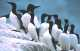Making Thumbnails (Complete) This example shows how to manually make a number of thumbnail images from an original. It also shows how you can manually set the size of your Canvas.
 1Creating the Canvas

We will need a canvas so we create it here.

[C#] ```Canvas canvas = new Canvas();```

[Visual Basic] ```Dim canvas As New Canvas()```

 2Creating the image

We create the image and draw options. We set the ImageFit parameter to AspectRatio. This will ensure that the image is scaled down to the Canvas size, with its aspect ratio preserved. We also calculate the aspect ratio of the image. We will use it later on to manually size the Canvas.

[C#] ```XImage image = XImage.FromFile(Server.MapPath("rez/pic.jpg")); DrawOptions drawOpts = new DrawOptions(); drawOpts.ImageFit = DrawOptions.ImageFitType.AspectRatio; double ratio = (double)image.Height / (double)image.Width;```

[Visual Basic] ```Dim image As XImage = XImage.FromFile(Server.MapPath("rez/pic.jpg")) Dim drawOpts As New DrawOptions() drawOpts.ImageFit = DrawOptions.ImageFitType.AspectRatio Dim ratio As Double = CDbl(image.Height) / CDbl(image.Width)```

 3Making the large thumbnail

We set manually the width and height of the Canvas. The width of the Canvas will be 240 pixels (this is the width of our large thumbnail) and we scale the height so that the image fits nicely into the Canvas without getting stretched. We draw the image precisely into the Canvas and then save it out. We call Clear() to reset the Canvas status and dimensions.

[C#] ```canvas.Width = 240; canvas.Height = 240 * ratio; canvas.DrawImage(image, drawOpts); canvas.SaveAs(Server.MapPath("Making_Thumbnails_Complete_6_large.jpg")); canvas.Clear();```

[Visual Basic] ```canvas.Width = 240 canvas.Height = 240 * ratio canvas.DrawImage(image, drawOpts) canvas.SaveAs(Server.MapPath("Making_Thumbnails_Complete_6_large.jpg")) canvas.Clear()```

 4Making the medium thumbnail

We set manually the width and height of the Canvas. The width of the Canvas will be 160 pixels (this is the width of our medium thumbnail) and we scale the height so that the image fits nicely into the Canvas without getting stretched. We draw the image precisely into the Canvas and then save it out. We call Clear() to reset the Canvas status and dimensions.

[C#] ```canvas.Width = 160; canvas.Height = 160 * ratio; canvas.DrawImage(image, drawOpts); canvas.SaveAs(Server.MapPath("Making_Thumbnails_Complete_6_medium.jpg")); canvas.Clear();```

[Visual Basic] ```canvas.Width = 160 canvas.Height = 160 * ratio canvas.DrawImage(image, drawOpts) canvas.SaveAs(Server.MapPath("Making_Thumbnails_Complete_6_medium.jpg")) canvas.Clear()```

 5Making the small thumbnail

We set manually the width and height of the Canvas. The width of the Canvas will be 80 pixels (this is the width of our small thumbnail) and we scale the height so that the image fits nicely into the Canvas without getting stretched. We draw the image precisely into the Canvas and then save it out. We call Clear() to reset the Canvas status and dimensions.

[C#] ```canvas.Width = 80; canvas.Height = 80 * ratio; canvas.DrawImage(image, drawOpts); canvas.SaveAs(Server.MapPath("Making_Thumbnails_Complete_6_small.jpg")); ```

[Visual Basic] ```canvas.Width = 80 canvas.Height = 80 * ratio canvas.DrawImage(image, drawOpts) canvas.SaveAs(Server.MapPath("Making_Thumbnails_Complete_6_small.jpg")) ```

 6Input and Output

Sample input and output images are shown below.rez/pic.jpgMaking_Thumbnails_Complete_6_large.jpgMaking_Thumbnails_Complete_6_medium.jpgMaking_Thumbnails_Complete_6_small.jpg